# Spread of a data set - standard deviation & variance#### Everything You Need in One Place

Homework problems? Exam preparation? Trying to grasp a concept or just brushing up the basics? Our extensive help & practice library have got you covered.#### Learn and Practice With Ease

Our proven video lessons ease you through problems quickly, and you get tonnes of friendly practice on questions that trip students up on tests and finals.#### Instant and Unlimited Help

Our personalized learning platform enables you to instantly find the exact walkthrough to your specific type of question. Activate unlimited help now!

0/0
0/5
##### Examples
###### Lessons
1. Determining Population Standard Deviation VS. Sample Standard Deviation
The heights of students (in cm) in a class are: {148, 156, 160, 164, 164, 167, 171, 176, 180, 194}
1. Calculate the Population Standard Deviation and Variance.
2. Randomly pick 3 data values and calculate the Sample Standard Deviation.
3. Verify the results using statistics commands on your calculator.
2. Determining the Standard Deviations from Histograms
Without calculation, determine which set of data is likely to have:
• the greatest standard deviation
• the smallest standard deviation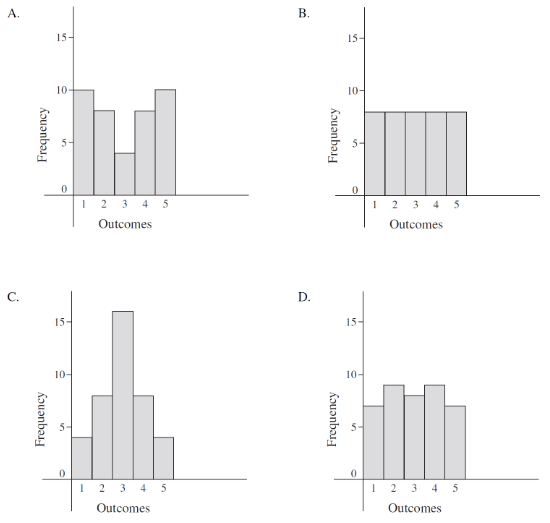1. Determining the Mean and the Standard Deviation from a Frequency Table
Determine the mean and the standard deviation for the population of scores in the following frequency table.

 score frequency 4 6 5 3 7 8 9 2

## Spread of data set: Standard deviation and variance

On this lesson we will focus on the topic of dispersion of data values from an expected result. The main concepts to learn are the standard deviation and variance, both related to describe the way how measurements, or outcomes, in a statistical experiment, or study, go away from the central tendency of the whole data set.

Before we get into these two concepts, we would like to explain dispersion with a little example study. Throughout the past century, technology development, curiosity and courage have tested humans ability to climb high mountains; thanks to this, people have been measuring Mount Everests height through the years obtaining different results. Some of the numbers (in meters) obtained are as follows:
• In 1954, Indian surveyors obtained a measurement of 8,848m.
• In 1975 Chinese surveyors obtained the same number of 8,848m.
• In 1999 American scientists used GPS technology and measured a height of 8, 850m.
• In 2005 Chinese surveyors re-measured the mountain and got a height of 8,844m.
• After the powerful earthquake that struck Nepal in 2015, the height of Mt. Everest is estimated to be 8,846m or 8,847m.

And so our data values are: 8848, 8848, 8850, 8844, 8846, 8847. The problem is, no one really knows what the real value is, we can only see how the real height of the mountain should be at an expected value, for which our best guess in the mean of all of the measured values so far.

This is where variance and standard deviation come to place; if we obtain the mean of the data values from the height of mount Everest, we obtain that our expected value is of 8,847.2m (the mean). But there is not a single measurement value in the data set that is exactly equal to this calculated number, the data set values are dispersed or spread from the expected one, the one that shows the central tendency of the data set.
The reasons why the data values are spread come from different kinds of errors in the measurement process; for example, not every team of surveyors measured the mountain with respect to sea level, also, not every single team used the exact same instruments or even the same metric system; hence, a variety of error types might have occured (systematic errors, human errors and random errors) producing an uncertainty on the true value of the height of the mountain. At the end, the data is inaccurate, and so, the measurement of this dispersion and how far this dispersion can go from the true value are what we study using the tools of standard deviation and variance.

#### What is a standard deviation

The standard deviation of a data set of values is the amount these values are spread from the mean. In simple words, when we find the standard deviation of a data set, we are calculating the amount of dispersion of the data values of the set from its expected value, which is the mean.

So, what does standard deviation mean in graphic representations of data distributions? By now we have seen that you can usually detect a central tendency, or a pattern followed by the values in a set to go towards the center of a data set, we talked about that in our last lesson; still, although all the values might have a tendency to a certain value, they will not all fall in the same one. This is how standard deviation comes to place, the standard deviation let us know how far away is each data value from the center; for a normal distribution (the most common type of distribution when studying random variables) this center is the mean.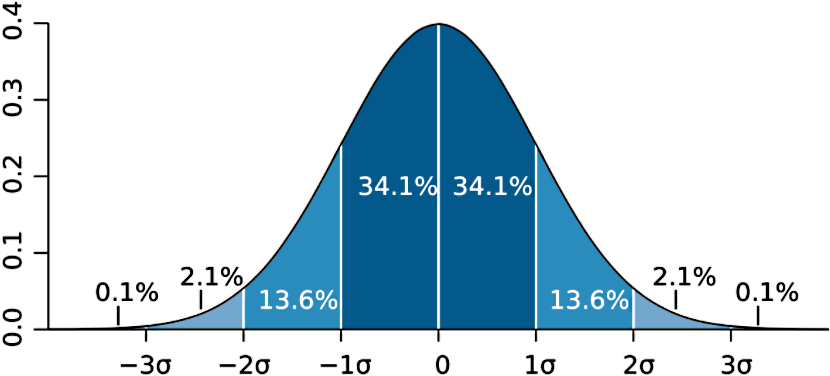In statistics, the standard deviation of a population is represented by the Greek letter sigma: $\sigma$.
And so, in figure 1 we can see that most of the data in a normal distribution (bell shaped distribution) is dispersed within one standard deviation from the mean, where the mean is the value of 0 (axis of symmetry of the bell shaped distribution).
As we have mentioned before, we will go into a detailed description of the normal distribution in a later lesson, and so, for now we will learn about the standard deviation for a population or a sample in general, no matter the shapes of distributions from the data sets in question.

#### How to calculate standard deviation

When calculating a standard deviation we need to know if we are looking into an entire statistical population or a sample of it. Therefore, there are two formulas to calculate standard deviation depending on the case:

We define standard deviation for a population ($\sigma$) as follows:

$\large \sigma = \sqrt{ \frac{(x_1-\mu)^{2} \, + \, ... \, + \,(x_n-\mu)^{2} }{n} }$

Where:
$\mu$ = mean
$n$ = total data values in the set.

In order to go through this formula and solve for the standard deviation of a population data set you need to follow the next steps:
1. Find the mean of the population ($\mu$= mean)
2. Subtract the mean to each data value in the population, then square the answer (squared differences $\,$$\, (x_{n} - \mu)^{2}$ ).
3. Calculate the average of the squared differences (add them all and divide by the total number of data values in the population set).
4. Take the square root of the answer you get from 3

On the other hand, the standard deviation of a sample is usually denoted with the letter s; and so, the formula for the sample standard deviation is:

$\large S = \sqrt{ \frac{(x_1-\bar{x})^{2} \, + \, ... \, + \,(x_n-\bar{x})^{2} }{n-1} }$

Where:
$\bar{x}$ = mean,
$n$ = total data values in the set.

The steps to follow to work through this formula for standard deviation are:
1. Find the mean of the population ($\bar{x}$= mean)
2. Subtract the mean to each data value in the population, then square the answer $\,$$\, (x_{n} - \bar{x})^{2}$ ).
3. Add all the squared differences and divide them by the total number of data values minus one.
4. Take the square root of the answer you get from 3

Notice how as the amount of data values in a set increases, the standard deviation calculation extends too.
The part inside the square root in equations 1 and 2 is the variance of the population or the sample, respectively. In general, the square root of the variance is equal to the standard deviation of a data set. Before we continue onto the variance definition, let us work through a standard deviation example for the two types of standard deviations we just saw:

#### Example 1

The heights of students (in cm) in a class are: {148, 156, 160, 164, 164, 167, 171, 176, 180, 194}. Find the Population Standard Deviation:

Using the steps we described above for equation 1 (the standard deviation of a population) we know that we first have to obtain the mean of the data set:

$\large \mu = \frac{148+156+160+164+164+167+171+176+180+194}{10} = \frac{1680}{10} =168$

Then we subtract the mean from each single value in the set, and square the result to obtain the squared differences:

$(x_1 - \mu)^{2} = (148-168)^{2} = 400$

$(x_2 - \mu)^{2} = (156-168)^{2} = 144$

$(x_3 - \mu)^{2} = (160-168)^{2} = 64$

$(x_4 - \mu)^{2} = (164-168)^{2} = 16$

$(x_5 - \mu)^{2} = (164-168)^{2} = 16$

$(x_6 - \mu)^{2} = (167-168)^{2} = 1$

$(x_7 - \mu)^{2} = (171-168)^{2} = 9$

$(x_8 - \mu)^{2} = (176-168)^{2} = 64$

$(x_9 - \mu)^{2} = (180-168)^{2} = 144$

$(x_{10} - \mu)^{2} = (194-168)^{2} = 676$

We add all of the squared differences together and divide them by the total amount of data values in the set ($n=10$ for this case).

$\large \frac{\sum_{1}^{n=10} (x_n - \mu)^{2}} {10} = \frac{(x_1 - \mu)^{2} + (x_2 - \mu)^{2} \,+ \, ... \, + \, (x_{10} - \mu)^{2}} {10}$

$\large \frac{\sum_{1}^{n=10} (x_n - \mu)^{2}} {10} = \frac{400+144+64+16+16+1+9+64+144+676} {10} =\frac{1534}{10} =153.4$

And now we just take the square root of the result we obtained above to finally find the population standard deviation:

$\sigma = \sqrt{\frac{\sum_{1}^{n=10} \enspace(x_n - \mu)^{2}}{10} } =\sqrt{153.4} =12.385$

Now, using the same data set, randomly pick 3 data values and calculate the standard deviation of this sample:
So we pick the values of 148, 164 and 176. For this question we use equation 2 (sample standard deviation formula), and so we start by obtaining the mean of the values in the sample:

$\large \mu = \frac{148+164+176} {3} = \frac{488}{3} =162.\overline{66}$

And so, using equation 2 we obtain the sample standard deviation (on this case we can summarize the steps since there are just a few values to use on the calculation):

$\large S = \sqrt{\frac{(x_1-\bar{x})^{2} \, + \, .... \, + \, (x_n - \bar{x})^{2}} {n-1}} = \sqrt{\frac{(148-162.\overline{66})^{2} \, + \, (164-162.\overline{66})^{2} + (176-162.\overline{66})^{2} } {3-1} }$

$\large S = \sqrt{\frac{215.092 +1.779+177.796} {3-1}} = \sqrt{\frac{394.667 } {2}} =\sqrt{197.333} =14.05$

Now that you have seen the difference between the population and sample standard deviation, let us learn about variance.

#### What is variance in statistics

The variance provides a measure of how much the data is spread from the mean of a data set, when comparing the variance vs standard deviation, the standard deviation refers to the spread of each particular data value, while the variance is the overall spread of the data from the mean. Therefore, if a data set contains values that are all the same, the variance will be zero, while data set values with high magnitude differences would form a set with a high variance; simply said, the larger the variance of a data set is, the larger the range of the data set. Variance builds off of the standard deviation. Youll find that once you find the standard deviation of a question, you can then easily find the variation.

Using the standard deviation definition for a population, we can simply define variance as:

$variance = \sigma^{2}$

In general, the variance is equal to the standard deviation square, which means that depending on the case, the variance formula will vary depending if you are obtaining the variance of a population or the variance of a sample group.

#### How to calculate variance

The population variance is calculated using equation 9, where is the population standard deviation symbol. Therefore, continuing with the data set from example 1: {148, 156, 160, 164, 164, 167, 171, 176, 180, 194}. We can calculate the population variance:

$variance = \sigma^{2} = (12.385)^{2} = 153.4$

Then, calculating variance of a sample is a very simple process! To find the sample variance we just need to square the number obtained as the sample standard deviation. Therefore the sample variance formula is:

$sample \; variance = S^{2}$

Were s is the standard deviation of a sample as shown in equation 2.
To finalize this lesson, let us work through a few examples where you will be asked to find standard deviation $\sigma$ or $s$ and calculate variance depending on the type of group being studied.

#### Example 2

Determining the Standard Deviations from Histograms
Without calculation, determine which set of data is likely to have:
• The greatest standard deviation
• The smallest standard deviation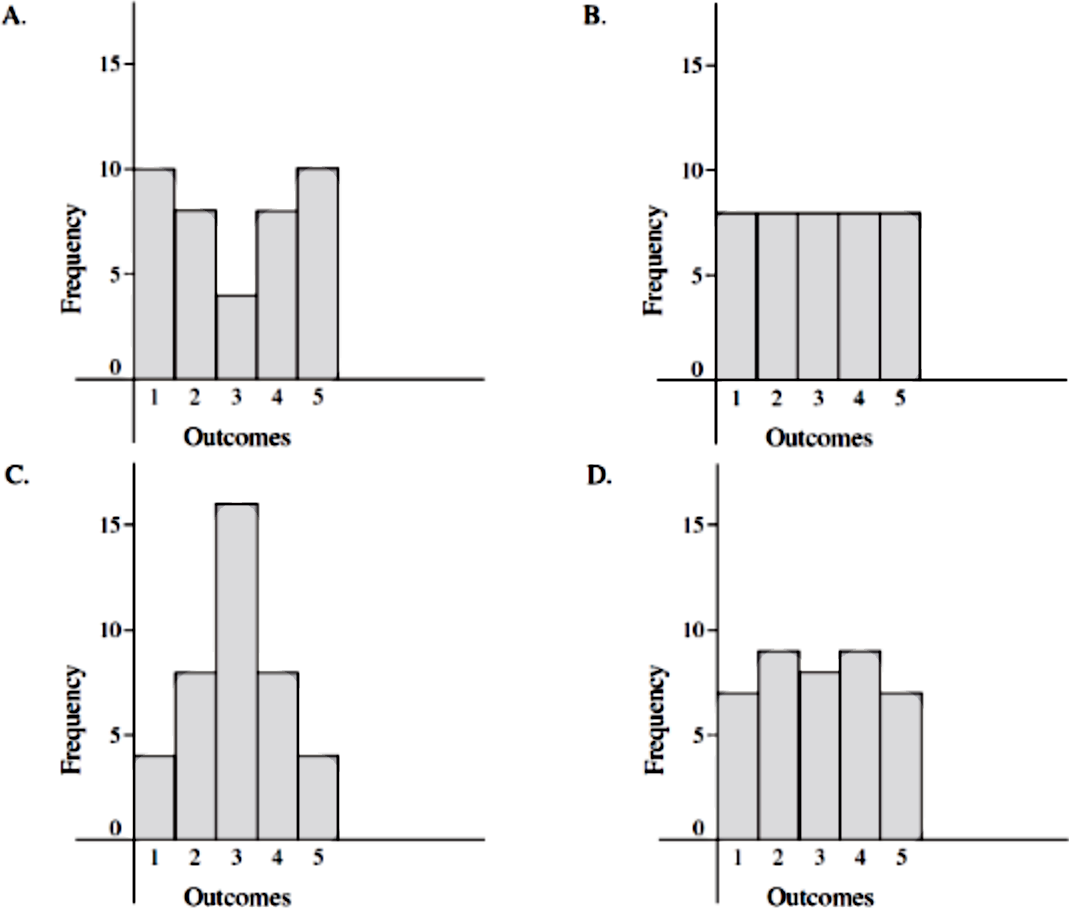Just by looking at the graphs we can right away observe that the histogram in part c has the lowest standard deviation since most of its data is concentrated in the center, and therefore, it means that the grand majority of its data points are close to the mean (they are not that dispersed from the central value).
On the other hand, identifying which of the histograms has the biggest standard deviation may be confusing; still, let us use the exact same logic as our statement above:
The bigger the dispersion of the data points in the graph, the biggest the standard deviation. Notice that all of the histograms are symmetric with a mean value of 3; therefore if we look at histogram a you can observe how this is the graph where the majority of the data points are farthest away from the mean value, and so, histogram a is the greatest standard deviation.

You can confirm this by calculating the standard deviation values. We have gathered the frequency distribution tables corresponding to each of the histograms in figure 2 so you can calculate the standard deviation of each histogram and check the answer above.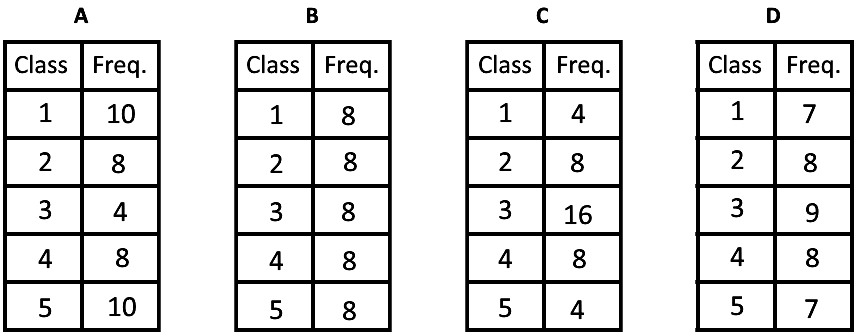#### Example 3

Determining the Mean and the Standard Deviation from a Frequency Table
Determine the mean and the standard deviation for the population of scores in the following frequency table.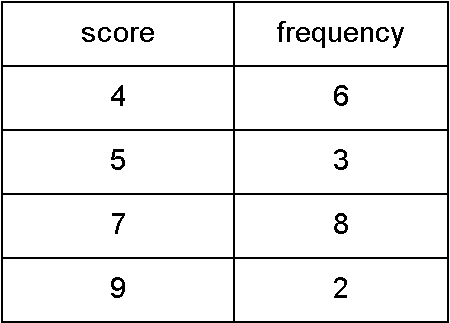From the table we can observe the data set is: {4, 4, 4, 4, 4, 4, 5, 5, 5, 7, 7, 7, 7, 7, 7, 7, 7, 9, 9}
Calculating the mean:

$\mu = \frac{4+4+4+4+4+5+5+5+7+7+7+7+7+7+7+7+9+9} {19} =\frac{113}{19} =5.95$

Now using the mean, and the standard deviation equation (equation 1) we calculate the standard deviation of the population. Start by computing the squared differences:

$(x_1 - \mu )^{2} = (4-5.95)^{2} = 3.8 \,$$\, 3.8 \times 6 = 22.8$

$(x_7 - \mu )^{2} = (5-5.95)^{2} = 0.9 \,$$\, 0.9 \times 3 = 2.7$

$(x_{10} - \mu )^{2} = (7-5.95)^{2} = 1.1 \,$$\, 1.1 \times 8 = 8.8$

$(x_{18} - \mu )^{2} = (9-5.95)^{2} = 9.3 \,$$\, 9.3 \times 2 = 18.6$

Notice we only obtained the square differences from $x_1, x_7, x_{10}$ and $x_{18}$; then we multiplied by the frequency of each of them.
The reason for that is that $x_1=x_2=x_3=x_4=x_5=x_6$, same goes for $x_7=x_8=x_9; x_{10}=x_{11}=x_{12}=x_{13}=x_{14}=x_{15}=x_{16}=x_{17}$ and $x_{18}=x_{19}$.
Then we add the results from the squared differences, divide that by the total amount of data values (in this case we have a total amount of 19 data values) and obtain its square root in order to obtain the standard deviation:

$\large \sigma = \sqrt{ \frac{ \sum_{1}^{n=19} \enspace (x_n - \mu)^{2}} {19}} = \sqrt{\frac{22.8+2.7+8.8+18.6}{19}} = \sqrt{\frac{52.9}{19}} = \sqrt{2.78} =1.669$

You can continue producing the variance analysis for this problem by using the variance equation shown in equation 10, we recommend you to do this computation for practice.

***

To finalize this lesson, we would like to recommend you to visit this website from Statistics Canada where the variance and standard deviation are explained in detail, including their characteristics; besides, this article on the variance and standard deviation, which includes a few more examples for you to practice.

This is it for our lesson of today, see you in the next one!
Standard Deviation measures the average distance data values are from the mean.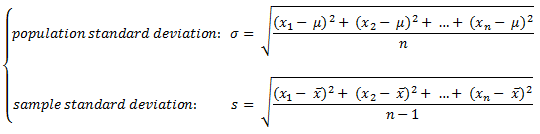note:
When the values in a data set are tightly bunched together, the standard deviation will be small.
When the values in a data set are spread apart, the standard deviation will be relatively large.

$variance=\sigma^2$